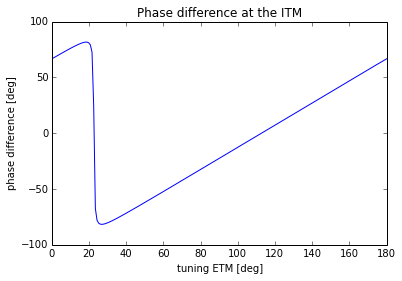# Example IPython notebook for using Finesse with PyKat

PyKat is a Python wrapper for the interferometer simulation Finesse. This notebook shows a simple example how PyKat can be used to call Finesse from Python in order to do some simple post-processing of the Finesse output.

The optical setup is an optical cavity, two amplitude detectors are used to measure the optical phases at the input mirror. Post-processing this output in Python we can plot the phase difference between the injected field and the circulating field. This difference becomes zero when the cavity is on resonance. Thus the phase difference presents a simple means to find the cavity resonance, for example with a root finding algorithm.

The notebook was started using 'ipython notebook --pylab inline'. You can download the notebook file: pykat_example1.ipynb

First we import the main bits from PyKat:

In :
from pykat import finesse
from pykat.commands import *

                                              ..-
PyKat 0.3.1           _                  '(
\.|\.__...-""""-_." )
..+-----.._        /  '             .-'
. '            :      7/* _/._\    \   (
(        '::;;+;;:      -"' =" /,"" ) /
L.        \:::a:f            c_/     n_'
..--...___.  .    ,
^-....____:   +.      www.gwoptics.org/pykat



Next we can create a 'kat' object and parse some Finesse source code to fill it:

In :
kat = finesse.kat()
kat.verbose = False
kat_code = """
l psl 1.0 0 npsl
s s1 0 npsl nITM1

m1 ITM 0.02 0 0 nITM1 nITM2
attr ITM Rc -2500
s s_cav 5000 nITM2 nETM1
m1 ETM 0.001 0 0 nETM1 nETM2
attr ETM Rc 2700
cav c1 ITM nITM2 ETM nETM1

yaxis deg
phase 0
"""

In :
kat.parseKatCode(kat_code)
kat.maxtem=0
kat.parseKatCode("xaxis ETM phi lin 0 180 200")


The following commands run the Finesse simulation and read the results into the variable 'out', followed by the calculation of the phase difference.

In :
out = kat.run()
rel_phase=(out["carr2"]-out["carr3"]-90)%360-180


And finally we plot the result:

In :
plot(out.x,rel_phase)
title(r'Phase difference at the ITM')
xlabel('tuning ETM [deg]')
ylabel('phase difference [deg]')

Out:
<matplotlib.text.Text at 0x11165a910>This is an example of a simple task that benfits from the interactive enviroment provided by iPython. More complex tasks are often easier to implement using python script files which can be run from the iPython console.